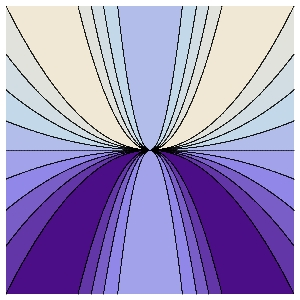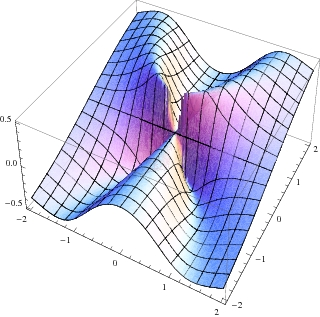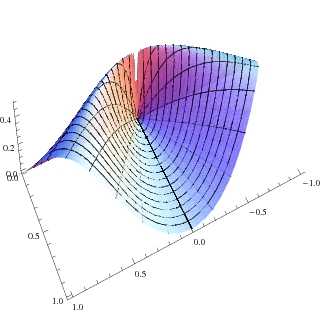Math 21a, Fall 2007
Exhibit page Math 21a 07, Multivariable Calculus
Scary: discontinuous f continuous on each line
 The function ``` f(x,y) = x2 y/(x4+y2) ``` is discontinuous at (0,0) but on each line through the origin, the function is continuous.The contour map of f. Level curves of different values meet at the origin. The graph of f shows the mountain rist, y=x2 on which the limit is 1/2 On every line through the origin, f is continuous: the limit is 0.